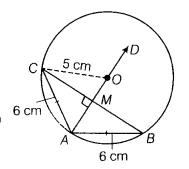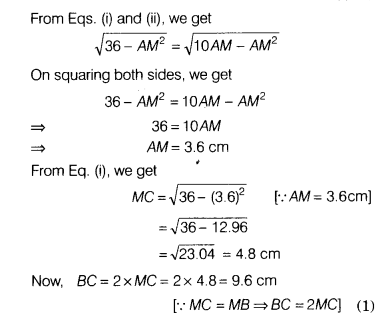# In a circle of radius 5 cm, AB and AC are two equal chords, such that, AB = AC = 6 cm

In a circle of radius 5 cm, AB and AC are two equal chords, such that, AB = AC = 6 cm. Find the length of the chord BC.

Let 0 be the centre of a circle and AD be the bisector of ∠BAC.Then, AD is the perpendicular bisector of BC and passes through the centre O. Join CO.
Let AD meets SC at M.
Then, MB = CM
In right angled ∆AMC,